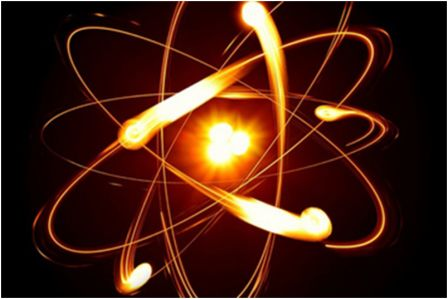/TOPOLOGICAL DIPOLE FIELD THEORY# TOPOLOGICAL DIPOLE FIELD THEORY

Germany– Patrick Linker, an Independent researcher and a student at Technische Universität Darmstadt in Germany is working on a topological dipole field theory. His personal interests are on Mechanics and Quantum field theory. Even though he studied mechanics at his university where he especially learnt the physical backgrounds of mechanical engineering, he has a large expertise in physics (especially Quantum physics literature). He adores the way how some important and useful equations are derived from a few fundamental assumptions. He thinks that even though quantum physics has achieved so much, it is yet not complete. There are still so many phenomena’s that cannot be described by current physical models.

Physics describes the foundation of nature. One of his childhood dreams was flying into space. But his bigger dream was to publish a scientific literature. He has succeeded to publish his new theory called  in an online journal called “The Winnower” which offers open peer review. This peer-review system is non-traditional peer review system where everyone registered on the webpage can give reviews.

[pullquote]Topological quantum field theory, as he explains, is a new Quantum field theory that extends Quantum electrodynamics. [/pullquote]

Topological quantum field theory, as he explains, is a new Quantum field theory that extends Quantum electrodynamics. While Quantum electrodynamics is a theory that describes the interaction of light with matter, Topological Dipole Field Theory describes more.

For example, self-interactions of photons are predicted by his theory. In other quantum field theories, self-interactions are proposed only for gluons (strong force carriers), electroweak bosons and the Higgs boson. These additional physical interactions are motivated from the assumption of his research paper that charged elementary particles can carry an additional degree of freedom: the intrinsic dipole moment. This additional degree of freedom modifies the interaction of matter with light. Topological Dipole Field Theory extends the electromagnetic field strength tensor by a topological term. Quantum electrodynamics is a theory with very high agreement with experiments, but still some differences were observed between experimental and theoretical results.

His theory remembers on electrodynamics with a coupling of the electromagnetic field to an electric dipole.  However, the theory has a dipole moment which has a topological nature.  The additional dipole moment is regarded as a geometric quantity, the intrinsic curvature.

This is an intrinsic curvature because it is added to the ordinary electromagnetic field strength tensor which is a curvature of a fiber bundle mathematically. But to obtain a topological quantity, the theory must be independent on this geometric quantity. If this is the case, the theory is called topological. Since Topological Dipole Field Theory is independent on the magnitude of this intrinsic dipole moment (but it is coupling on this field) Topological field theory is a topological quantum field theory.

This is an intrinsic curvature because it is added to the ordinary electromagnetic field strength tensor which is a curvature of a fiber bundle mathematically. But to obtain a topological quantity, the theory must be independent on this geometric quantity. If this is the case, the theory is called topological. Since Topological Dipole Field Theory is independent on the magnitude of this intrinsic dipole moment (but it is coupling on this field) Topological field theory is a topological quantum field theory.

This generalization of quantum electrodynamics by topological quantum fields is a model for describing a hypothetical intrinsic dipole moment of a charged elementary particle. His paper shows that the topological term of the action is similar to the abelian Chern-Simons theory. The photon field strength tensor of quantum electrodynamics is coupled to an additional dipole moment which is of purely topological nature.